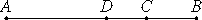# Proposition 46

The side of a rational plus a medial area is divided at one point only.
X.40

Let AB be the side of a rational plus a medial area divided at C, so that AC and CB are incommensurable in square and let the sum of the squares on AC and CB be medial, but twice the rectangle AC by CB rational.

I say that AB is not so divided at another point.If possible, let it be divided at D also, so that AD and DB are also incommensurable in square and the sum of the squares on AD and DB is medial, but twice the rectangle AD by DB rational.

X.26

Since, then, that by which twice the rectangle AC by CB differs from twice the rectangle AD by DB is also that by which the sum of the squares on AD and DB differs from the sum of the squares on AC and CB, while twice the rectangle AC by CB exceeds twice the rectangle AD by DB by a rational area, therefore the sum of the squares on AD and DB also exceeds the sum of the squares on AC and CB by a rational area, though they are medial, which is impossible.

Therefore the side of a rational plus a medial area is not divided at different points, therefore it is divided at one point only.

Therefore, the side of a rational plus a medial area is divided at one point only.

Q.E.D.

## Guide

This proposition is not used in the rest of the Elements.